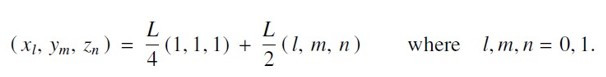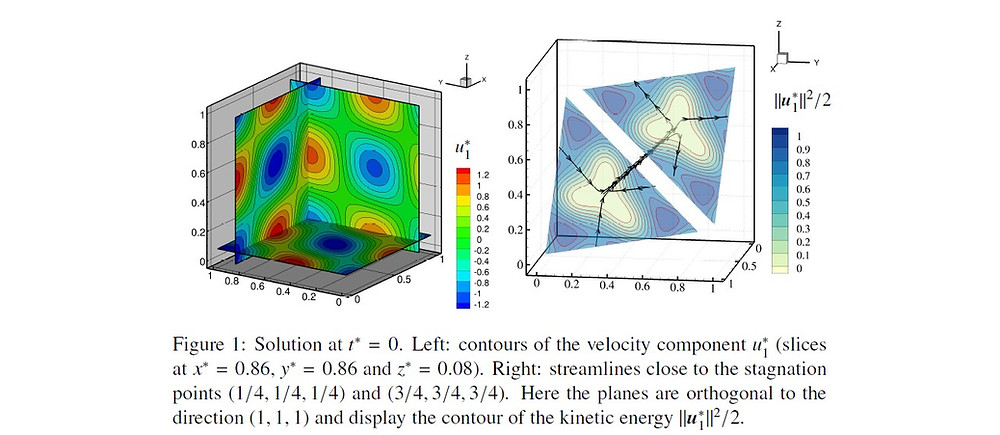## Test 16

Decay of three-dimensional vortices

(M. Antuono)

(Download full test case data files here: SPHERIC_TestCase16.zip)

### Introduction

The unsteady tri-periodic laminar solutions of the Navier-Stokes equations described in Antuono (2020) are considered as possible benchmarks. These represent fully three-dimensional flows, since all the velocity components depend non-trivially on all three coordinate directions, and can be regarded as an extension in three-dimensions of the bi-periodic vortex solution of Taylor (1923). For low and moderate Reynolds numbers these solutions can be used for benchmarking numerical codes while, for larger Reynolds numbers, they may be adopted to study the onset of flow instabilities and the eventual transition to turbulence.

### Flow phenomena

• laminar viscous flow

• fully three-dimensional

• tri-periodic

• transition to turbulence for high Reynolds numbers

### Geometry

The geometry is a three-dimensional cube with periodic boundary conditions (namely, a three-dimensional Torus). Specifically, the domain is D = [0, L]3 where L denotes the length of one side of the cube.

### Boundary conditions

Periodic conditions are assigned on all facets of the cube.

### Initial conditions

The initial conditions are obtained by imposing t = 0 in the analytic solutions for the fluid velocity, u = (u,v,w). At each time instant, the pressure field can be obtained straightforwardly from the solution for u through the relation belowwhere p0 represents an arbitrary constant and ρ0 is the reference density. The analytic solutions for the velocity field are classified according to the sign of the helicity (namely, the scalar product between velocity and vorticity). In particular we use the subscript ‘1’ for the solution with positive helicity (namely u·ω > 0) and the subscript ‘2’ for the solution with negative helicity (i.e. u·ω < 0). We have:where k = 2π/L is the wave number, L is the length of one side of the cube, ν is the kinematic viscosity and U0 is the reference velocity. The Reynolds number is defined as Re = U0L/ν. The analytic solutions are renormalized so that the average kinetic energy per unit of mass satisfies the following relation:and (0) = U02/2. The maximum absolute velocity is attained at the initial time (for example, at the origin) and corresponds to Umax= √(8/3)U0. The vorticity field is obtained through the solution of the velocity field as follows:Both solutions have eight stagnation points at the positions:### Discretisation

The initial discretization may be a uniform Cartesian lattice or, for SPH solvers, any regular particle distribution. In Antuono (2020) the numerical outputs were obtained through a Finite Volume solver with 323 nodes displaced on a regular Cartesian grid. For Re = 50 the numerical solution was in good agreement with the analytical solution while for larger values (e.g. Re = 1000) the onset of instabilities was observed.

### Results specification

Comparisons with the analytic solutions described in ”Initial conditions”. In particular:

• time decay of the average kinetic energy per unit of mass for different Reynolds numbers.

• convergence analysis on for a fixed Reynolds number

• prediction of particle trajectories

• contour maps of the velocity and pressure/vorticity fields.

See figures 1, 2 and 3 as possible examples.

### Results format

Results are in the form of analytic expressions (see the section ”Initial conditions”)

### Benchmark results

Numerical solutions obtained through a Finite-Volume solver are available in Antuono (2020).### SPH Publications using this Case

If you have published results for this case, please email the webmaster to have your papers added.

### References

• Antuono, M. (2020). Tri-periodic fully three-dimensional analytic solutions for the Navier–Stokes equations. Journal of Fluid Mechanics, 890, A23. doi:10.1017/jfm.2020.126.

• Taylor, G. I. (1923) LXXV. On the decay of vortices in a viscous fluid. Phil. Mag. 46, 671–674.